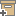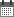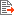ניהול
קהילה:
אסיף מאגר המחקר החקלאי
Semianalytical solution for dual-probe heat- pulse applications that accounts for probe radius and heat capacityBack to searchPrevious item
Next item
Year:
2012
Source of publication :
Authors :
קמאי, תמיר
;
.
Volume :
11
Co-Authors:
Knight, J.H., Faculty of Science and Technology, Queensland Univ. of Technology, Brisbane, QLD 4001, Australia
Kluitenberg, G.J., Dep. of Agronomy, Kansas State Univ., Manhattan, KS 66506, Australia
Kamai, T., Dep. of Land, Air and Water Resources, Univ. of California, Davis, CA 95616, Australia
Hopmans, J.W., Dep. of Land, Air and Water Resources, Univ. of California, Davis, CA 95616, Australia
Facilitators :
From page:
To page:
(
Total pages:
1
)
Abstract:
The dual-probe heat-pulse (DPHP) method is useful for measuring soil thermal properties. Measurements are made with a sensor that has two parallel cylindrical probes: one for introducing a pulse of heat into the soil (heater probe) and one for measuring change in temperature (temperature probe). We present a semianalytical solution that accounts for the finite radius and finite heat capacity of the heater and temperature probes. A closed-form expression for the Laplace transform of the solution is obtained by considering the probes to be cylindrical perfect conductors. The Laplacedomain solution is inverted numerically. For the case where both probes have the same radius and heat capacity, we show that their finite properties have equal influence on the heat-pulse signal received by the temperature probe. The finite radius of the probes causes the heat-pulse signal to arrive earlier in time. This time shift increases in magnitude as the probe radius increases. The effect of the finite heat capacity of the probes depends on the ratio of the heat capacity of the probes (C0) and the heat capacity of the soil (C). Compared with the case where C0/C = 1, the magnitude of the heat-pulse signal decreases (i.e., smaller change in temperature) and the maximum temperature rise occurs later when C0/C > 1. When C0/C < 1, the magnitude of the signal increases and the maximum temperature rise occurs earlier. The semianalytical solution is appropriate for use in DPHP applications where the ratio of probe radius (α0) and probe spacing (L) satisfies the condition that a0/L ≤ 0.11. © Soil Science Society of America.
Note:
Related Files :
Laplace-domain solution
measurement method
Semi-analytical solution
Soils
soil temperature
עוד תגיות
תוכן קשור
More details
DOI :
Article number:
Affiliations:
Database:
סקופוס
Publication Type:
מאמר
;
.
Language:
אנגלית
Editors' remarks:
ID:
20990
Last updated date:
02/03/2022 17:27
Creation date:
16/04/2018 23:40Scientific Publication
Semianalytical solution for dual-probe heat- pulse applications that accounts for probe radius and heat capacity
11
Knight, J.H., Faculty of Science and Technology, Queensland Univ. of Technology, Brisbane, QLD 4001, Australia
Kluitenberg, G.J., Dep. of Agronomy, Kansas State Univ., Manhattan, KS 66506, Australia
Kamai, T., Dep. of Land, Air and Water Resources, Univ. of California, Davis, CA 95616, Australia
Hopmans, J.W., Dep. of Land, Air and Water Resources, Univ. of California, Davis, CA 95616, Australia
Semianalytical solution for dual-probe heat- pulse applications that accounts for probe radius and heat capacity
The dual-probe heat-pulse (DPHP) method is useful for measuring soil thermal properties. Measurements are made with a sensor that has two parallel cylindrical probes: one for introducing a pulse of heat into the soil (heater probe) and one for measuring change in temperature (temperature probe). We present a semianalytical solution that accounts for the finite radius and finite heat capacity of the heater and temperature probes. A closed-form expression for the Laplace transform of the solution is obtained by considering the probes to be cylindrical perfect conductors. The Laplacedomain solution is inverted numerically. For the case where both probes have the same radius and heat capacity, we show that their finite properties have equal influence on the heat-pulse signal received by the temperature probe. The finite radius of the probes causes the heat-pulse signal to arrive earlier in time. This time shift increases in magnitude as the probe radius increases. The effect of the finite heat capacity of the probes depends on the ratio of the heat capacity of the probes (C0) and the heat capacity of the soil (C). Compared with the case where C0/C = 1, the magnitude of the heat-pulse signal decreases (i.e., smaller change in temperature) and the maximum temperature rise occurs later when C0/C > 1. When C0/C < 1, the magnitude of the signal increases and the maximum temperature rise occurs earlier. The semianalytical solution is appropriate for use in DPHP applications where the ratio of probe radius (α0) and probe spacing (L) satisfies the condition that a0/L ≤ 0.11. © Soil Science Society of America.
Scientific Publication
You may also be interested in